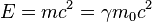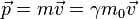# What specific momentum should be used in the matter wave?

• I
• LarryC

#### LarryC

According to de Broglie's hypothesis, the matter wavelength could be described by lamda=h/p. But which momentum, the classical p=mv, or the relativistic one p=mv(1-v^2/c^2)^(-1/2) should be used in this equation? Additionally, an even more confusing issue to me is regarding the other relation E=hf. I'm not sure whether the energy on the left-hand side is the kinetic energy K=mc^2(gamma-1) or the total energy E=gamma*mc^2.

According to de Broglie's hypothesis, the matter wavelength could be described by lamda=h/p. But which momentum, the classical p=mv, or the relativistic one p=mv(1-v^2/c^2)^(-1/2) should be used in this equation? Additionally, an even more confusing issue to me is regarding the other relation E=hf. I'm not sure whether the energy on the left-hand side is the kinetic energy K=mc^2(gamma-1) or the total energy E=gamma*mc^2.

de Broglie relations are used both in relativistic and non relativistic quantum mechanics; meaning thereby that when velocities are small compared to c, vel of light one can use non relativistic expression for momentum and if velocities are comparable to c ,the relativistic momentum may be used.

Using two formulas from special relativity, one for the relativistic momentum and one for the energywhere m0 is rest mass of the particle .

see for details: https://en.wikipedia.org/wiki/Matter_wave#Historical_context

Yep I understand this. But what confuses me is the huge difference between the classical theory and the relativity when calculating the frequency. I mean, for example, consider a particle moving at a low speed. Then f=E/h gives either f=mc^2/h from relativistic perspective or f=mv^2/(2h) in classical mechanics, which leads to a significant difference. So how to explain this?

Last edited by a moderator:
Yep I understand this. But what confuses me is the huge difference between the classical theory and the relativity when calculating the frequency. I mean, for example, consider a particle moving at a low speed. Then f=E/h gives either f=mc^2/h from relativistic perspective or f=mv^2/(2h) in classical mechanics, which leads to a significant difference. So how to explain this?
i do not think that any classical perspective of matter waves can be picturised -as the duality predefines the quantum behavior of particles in microworld - the classical approach fell through in explaining the black body radiation intensity along with other experimental results like Compton scattering of x-rays or absorption spectra, Davisson Germer diffraction of electrons expt etc.,
so if we wish to compare with classical energy and momentum we will be making a serious error. the domain of Planck's constant is the quantum region-though sometimes we may calclate waves associated with moving bodies classically just to show that its not meaningful.

Yep I understand this. But what confuses me is the huge difference between the classical theory and the relativity when calculating the frequency. I mean, for example, consider a particle moving at a low speed. Then f=E/h gives either f=mc^2/h from relativistic perspective or f=mv^2/(2h) in classical mechanics, which leads to a significant difference. So how to explain this?
That huge difference appears because with a slow-moving object the difference between the total energy and the kinetic energy is also huge.
At low speeds the classical ##p=mv## is a good approximation to the relativistic ##p=\gamma{m}_0v##, and the classical ##E_k=(mv^2)/2## is a good approximation to the relativistic ##E_k=(\gamma-1)m_0c^2## - but there is no remotely close classical approximation to the relativistic total energy. So the moral of the story is that if you want a classical approximation that works at low speeds, you work with formulas that depend on the momentum or the kinetic energy, not the total energy.

You also have to watch out for the relativistic approximation ##E_k\approx{E}_{tot}## for very relativistic speeds (##\gamma>>1##), which will lead you into confusion about which energy you're using in a relativistic formula, might even tempt you to try using the classical ##E_k## where it's inappropriate.

Yep I understand this. But what confuses me is the huge difference between the classical theory and the relativity when calculating the frequency. I mean, for example, consider a particle moving at a low speed. Then f=E/h gives either f=mc^2/h from relativistic perspective or f=mv^2/(2h) in classical mechanics, which leads to a significant difference. So how to explain this?
This difference you already have in classical particle theory. The reason is that in the relativistic case it is convenient to define the energy of the particle as
$$E=\frac{m c^2}{\sqrt{1-v^2/c^2}}.$$
If you expand the square root in powers of ##v^2/c^2##, i.e., for small velocities ##|v|\ll c^2## you find
$$E=m c^2 + \frac{m}{2} v^2 + \cdots$$
This means the energy is counted from the rest energy ##E_0=m c^2##. The reason for this choice of the energy-zero level is that with this choice the energy builds together with momentum a four-vector, the socalled four-momentum,
$$(p^{\mu})=\begin{pmatrix} E/c \\ \vec{p} \end{pmatrix},$$
where
$$\vec{p}=\frac{m \vec{v}}{\sqrt{1-\vec{v}^2/c^2}}.$$
This, however, doesn't invalidate the de Broglie relation for a non-relativistic particle. If you want to get this you have to remember that you can always multiply a wave function with a phase factor. So if ##\Phi(x)## is a relativistic "wave function" (e.g., for a scalar particle), then it's related in the non-relativistic limit to the Schrödinger wave function ##\psi##
$$\Phi(t,\vec{x}) \simeq \exp \left (-\frac{m c^2 t}{\hbar} \right) \psi(\vec{x}).$$
Then the de Broglie relations between the frequencies are (approximately) correct for the non-relativistic case ##|\vec{v}|\ll c## or, equivalently, ##|c \vec{p}| \ll m c^2##, where ##\vec{p}## is a typical momentum of the wave packets described by the wave functions (supposed you have such a case of a wave function at all).